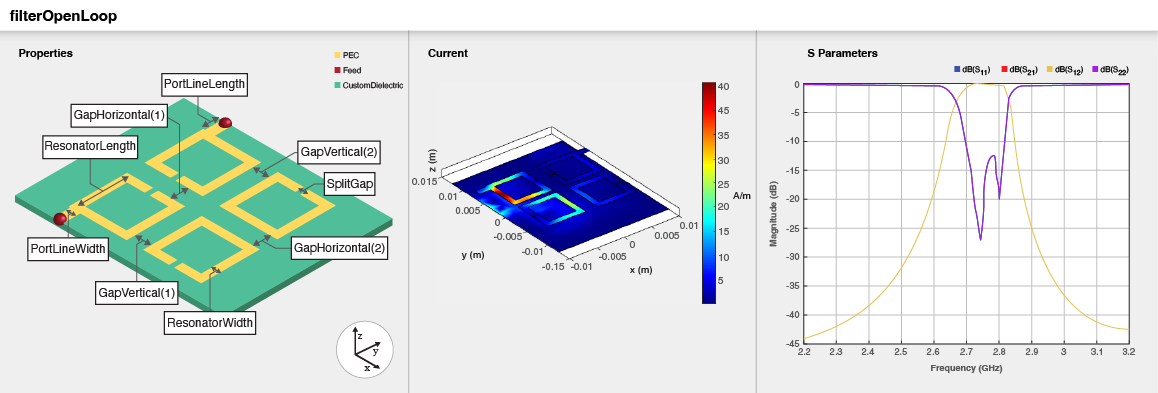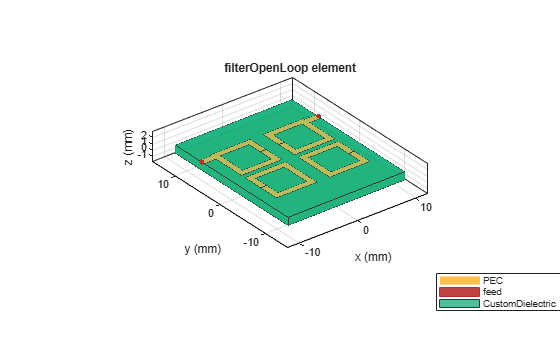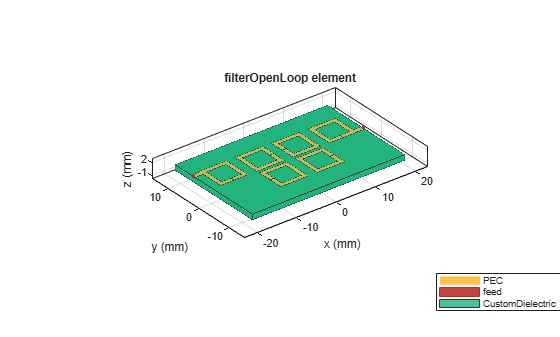# filterOpenLoop

Create open-loop band pass filter in microstrip form

Since R2023a

## Description

Use the `filterOpenLoop` object to create an open-loop bandpass filter in the microstrip form.## Creation

### Syntax

``filter = filterOpenLoop``
``filter = filterOpenLoop(Name=Value)``

### Description

example

````filter = filterOpenLoop` creates an open-loop bandpass filter with default properties for a cutoff frequency of 2.75 GHz.```

example

````filter = filterOpenLoop(Name=Value)` sets Properties using one or more name-value arguments. For example, `filterOpenLoop(ResonatorLength=0.008)` creates an open-loop bandpass filter with resonator length of 8 cm. Properties not specified retain their default values.```

## Properties

expand all

Number of poles in the filter, specified as `4`, `6`, or `8`.

Example: `filter = filterOpenLoop(NumPoles=8)`

Data Types: `double`

Length of resonator in meters, specified as a scalar.

Example: `filter = filterOpenLoop(ResonatorLength=0.009)`

Data Types: `double`

Width of resonator in meters, specified as a scalar.

Example: `filter = filterOpenLoop(ResonatorWidth=0.002)`

Data Types: `double`

Length of the split in the resonator in meters, specified as a scalar.

Example: `filter = filterOpenLoop(SplitGap=0.0001)`

Data Types: `double`

Horizontal gaps between the open-loop resonators of a quadruplet in meters, specified as one of these:

• scalar

• two-element vector when `NumPoles` is `2` or `4`

• four-element vector when `NumPoles` is `8`

Example: `filter = filterOpenLoop(GapHorizontal=[0.0033 0.0044])`

Data Types: `double`

Vertical gaps between the open-loop resonators of a quadruplet in meters, specified as one of these:

• scalar

• two-element vector when `NumPoles` is `2` or `4`

• four-element vector when `NumPoles` is `8`

Example: `filter = filterOpenLoop(GapVertical=[0.0009 0.0008])`

Data Types: `double`

Offset of the input and output feed lines in meters, specified as a scalar or a two-element vector. If you specify a scalar value, then input and output lines are offset by the same value. If you specify a two-element vector, the first element represents the offset for the input line and the second element the offset for the output line.

Example: `filter = filterOpenLoop(FeedOffset=[0.0076 0.0096])`

Data Types: `double`

Spacing between the quadruplet and input and output resonators in meters, specified as a scalar or a two-element vector. This property is enabled only when you set `NumPoles` to 6.

Example: ```filter = filterOpenLoop(CoupledResonatorGap=[0.0032 0.0042])```

Data Types: `double`

Spacing between the two quadruplets in meters, specified as a scalar. This property is enabled only when you set `NumPoles` to 8.

Example: `filter = filterOpenLoop(QuadrupletGap=0.00275)`

Data Types: `double`

Offset the center of right side quadruplet along Y-axis in meters, specified as a scalar. This property is enabled only when you set `NumPoles` to 8

Example: `filter = filterOpenLoop(QuadrupletOffset=0.3)`

Data Types: `double`

Length of the input and the output line in meters, specified as a positive scalar.

Example: `filter = filterOpenLoop(PortLineLength = 0.0035)`

Data Types: `double`

Width of the input and the output line in meters, specified as a positive scalar.

Example: `filter = filterOpenLoop(PortLineWidth = 0.001135)`

Data Types: `double`

Height from the ground plane to the filter in meters, specified as a positive scalar.

Example: `filter = filteropenLoop(Height = 0.00096)`

Data Types: `double`

Width of the ground plane in meters, specified as a positive scalar.

Example: `filter = filteropenLoop(GroundPlaneWidth = 0.00096)`

Data Types: `double`

Type of dielectric material used as a substrate, specified as a `dielectric` object. The dielectric material in a `filterOpenLoop` object has these default properties: `(Name='CustomDielectric',EpsilonR=10.8,LossTangent=0.0001,Thickness=1.27e-3)`

Example: `d = dielectric("FR4");` `coupler = filterOpenLoop(Substrate=d)`

Type of metal used in the conducting layers, specified as a `metal` object. The metal used in the conducting layers of `filterOpenLoop` object with default properties is PEC.

Example: `m = metal("Copper");` `coupler = filterOpenLoop(Conductor=m)`

Data Types: `string` | `char`

## Object Functions

 `charge` Calculate and plot charge distribution `current` Calculate and plot current distribution `dgs` Create defected ground structure of PCB element `feedCurrent` Calculate current at feed port `layout` Plot all metal layers and board shape `mesh` Change and view mesh properties of metal or dielectric in PCB component `shapes` Extract all metal layer shapes of PCB component `show` Display PCB component structure or PCB shape `sparameters` Calculate S-parameters for RF PCB objects

## Examples

collapse all

Create an open-loop filter with default properties.

`filter = filterOpenLoop`
```filter = filterOpenLoop with properties: NumPoles: 4 ResonatorLength: 0.0070 ResonatorWidth: 1.0000e-03 SplitGap: 1.0000e-04 GapHorizontal: 0.0022 GapVertical: 8.0000e-04 FeedOffset: 0.0066 PortLineWidth: 0.0011 PortLineLength: 0.0020 Height: 0.0013 GroundPlaneWidth: 0.0250 Substrate: [1x1 dielectric] Conductor: [1x1 metal] ```

View the filter.

`show(filter)`Create an open-loop filter with six poles and a different offset for the input and output lines.

`filter = filterOpenLoop(NumPoles=6,FeedOffset=[0.0066,0.001]);`

View the filter.

`show(filter);`Pozar, David M. Microwave Engineering. 4th ed. Hoboken, NJ: Wiley, 2012.

 Hong, Jia-Sheng, and M. J. Lancaster. Microstrip Filters for RF/Microwave Applications. Wiley, 2001.

 Pal Singh, Inder, Praveen Bhatt, Dinesh Bisht. "Design of Tunable Microstrip Bandpass Cascaded Quadruplet Filter". Advances in Computer Science and Information Technology (ACIST).Vol 2, Number 12, (July -September 2015) pp 11-14.

 Hong, Jia-Sheng, Michael J Lancaster. "Couplings of Microstrip Square, Open-Loop Resonators or Cross-Coupled Planar Microwave Filters". IEEE Transactions on Microwave Theory and Techniques.Vol 44, Number 12, (December 1996).